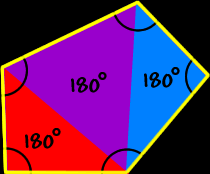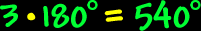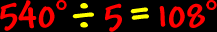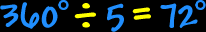# Polygons - Pentagons

Properties of pentagons, interior angles of pentagons

 Polygons:  Properties of PentagonsSum of the Interior Angles of a Pentagon:To find the sum of the interior angles of a pentagon, divide it up into triangles... There are three triangles...  Because the sum of the angles of each triangle is 180 degrees...  We getSo, the sum of the interior angles of a pentagon is 540 degrees.Regular Pentagons: The properties of regular pentagons:All sides are the same length (congruent) and all interior angles are the same size (congruent).To find the measure of the interior angles, we know that the sum of all the angles is 540 degrees (from above)...  And there are five angles...So, the measure of the interior angle of a regular pentagon is 108 degrees.The measure of the central angles of a regular pentagon:To find the measure of the central angle of a regular pentagon, make a circle in the middle...  A circle is 360 degrees around...  Divide that by five angles...So, the measure of the central angle of a regular pentagon is 72 degrees.